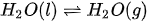# Problem: What is the boiling point water at the top of Mt Everest?The atmospheric pressure is 0.54 atm. The thermodynamic table of water at 25°C is shown below.SubstanceΔH°f (kJ/mol)S° (J/mol K)ΔG°fH2O(l)-285.870.0-237.1H2O(g)-241.8188.8-228.6

###### FREE Expert Solution

We will use the Clausius Clapeyron Equation

We are going to do the following steps:

Step 1: Calculate ΔH

Step 2: Calculate for boiling point

81% (330 ratings)###### Problem Details

What is the boiling point water at the top of Mt Everest?The atmospheric pressure is 0.54 atm. The thermodynamic table of water at 25°C is shown below.

 Substance ΔH°f (kJ/mol) S° (J/mol K) ΔG°f H2O(l) -285.8 70.0 -237.1 H2O(g) -241.8 188.8 -228.6